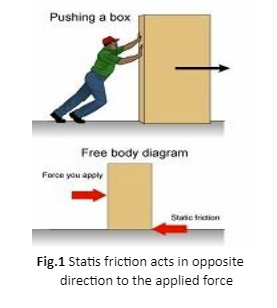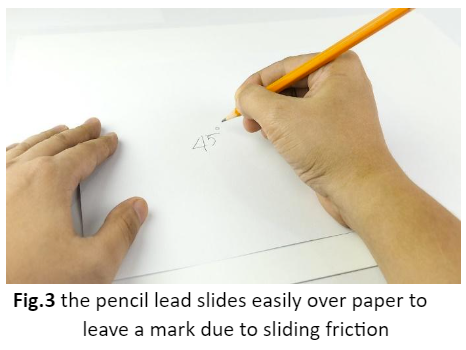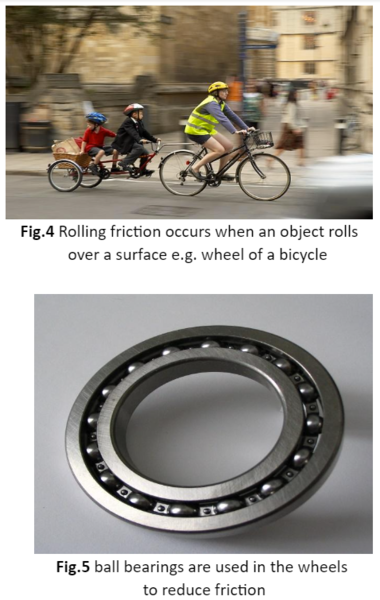• ### Browse All Lessons

##### Assign Lesson

Help Teaching subscribers can assign lessons to their students to review online!

 Tweet# Types of Friction

This lesson aligns with Next Generation Science Standards (NGSS) PS2.B

Introduction
Friction is the resistance offered by the surfaces that are in contact when they rub against each other. The frictional force is caused by the interaction between the microscopic bumps on the surfaces of objects when they are in contact. Friction has been categorized into four basic types that are static friction, sliding friction, rolling friction, and fluid friction. All four types of frictional force are different from each other. For instance, friction acts on the objects that are at rest named static friction and sliding friction occur when two solid surfaces slide against each other. In this article, we will learn about static friction, sliding friction, rolling friction, and fluid friction.

Types of Friction
Friction is a force that resists the motion of solid objects against each other. In general, four types of friction are mentioned below;
• Static Friction
• Sliding Friction
• Rolling Friction
• Fluid Friction

Static Friction
Static friction is defined as friction that acts on objects when they are at rest relative to each other.  Static friction resists the force that is applied to the object. When a small amount of force is applied to the object, the strength of the static friction becomes equal in the opposite direction which keeps the object at rest. If the force increases, the static friction may reach its maximum value at some points.

When the objects are at rest, we must use extra force to start the motion because of static friction.  if the applied force is less than the force of static friction, the stationary objects will not move. Therefore, we must apply a force greater than static friction to start the motion.A heavy box resting on the floor, difficult to lift and move, is a great example of static friction. Similarly, static friction plays an important role between your shoes and the trail when you are hiking in the woods. Other examples include skiing on the ice, a towel hanging on the rack, etc.Sliding Friction
Sliding friction is defined as the resistance that occurs between solid objects when they slide over each other. Sliding friction is weaker than static friction because it is easy to move the object that is already in motion than at rest.

For instance, when you stop a bicycle with hand brakes, the sliding friction between the rubber pads and metal rim of the wheel causes to slow down the motion of the wheel, and eventually stops it. Similarly, sliding friction occurs when you write with a pencil over paper.Rolling Friction
Rolling friction is a force of resistance that occurs when an object rolls across a surface. In general, rolling friction is less than sliding friction for similar materials and it provides less friction between the surfaces. Because of the weak nature of rolling friction, engineers who design bicycles, cars, and skateboards, use wheels that move easily. Therefore, ball bearings are used to reduce the friction between the wheels. These ball bearings are smooth steel balls lubricated with oil or grease that reduce friction by rolling between the moving parts of the vehicles and other machinery.Fluid Friction
A fluid is a substance or material that can flow easily such as water, oil, or air. Fluid friction is defined as the force of friction that acts on the objects which are moving through a fluid. Fluid friction is less than sliding friction. If you try to push the water with an open hand in a pool or a tub, you will feel the resistance of water against your hand, this type of resistance is known as fluid friction.

For instance, imagine you are sitting in a car that is moving in a forward direction. If you put your hand out the window, you will feel the force pushing your hand back as you move forward. This is fluid friction. The faster the moving object is, the greater the fluid friction affects its motion.

Summary
• Friction is a force of resistance that occurs between objects when they rub against each other.
• Static friction acts on objects that are at rest e.g. table lamp resting on the table.
• Sliding friction is defined as the frictional force that occurs between objects when they slide over each other.
• Rolling friction occurs when objects roll over a surface.
• Fluid friction is a frictional force that acts on the objects that are moving through a fluid.

Related Worksheets: